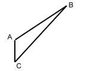# Find the sum of the vectors u and v if theta is the angle between them

DevilTemptationsAfter spending about an hour on this problem, I've become completely convinced that my math book is wrong in the answer it provides. Am I wrong or right? This is from the Harcourt Geometry and Discrete Mathematics Text.

Question:
Find the sum of the vectors u and v if theta is the angle between them.

Given:
u=3 (magnitude)
v=10 (magnitude)
theta=115 degrees

Obviously, the angles in a parallelogram equal to 360. This means the other vertexs are 65 degrees. Then I would use the cosine law to solve for the sum of the vectors. (I wish I could draw a diagram but I can't). So...

u+v^2=(10)^2+(3)^2-2(10)(3)Cos 65 degrees
therefore, u+v=9.1 (approx)

However, the back of the book says u+v=11.6

I've found that to get 11.6, instead of putting Cos 65, I'd have to put Cos 115 but that would not follow according to the laws. Another way is instead of finding the sum, I'd actually be finding the DIFFERENCE between the vectors.

Then I thought maybe I'm doing something wrong... because in the previous question, the angle between the vectors was 70 degrees. The angle between the vectors was not REALLY 70, instead it was divided into two angles and 70 degrees was one of them. Thus, an exterior angle from 70 which is 110 is given... so this proves the vertex opposite from it would be 70. Use Cosine law, I got the right answer.

However, this is an obtuse angle. There is obviously a different method but maybe I don't know it.

I'm confused :yuck:

Any ideas within the next hour or two would be appreciated...

Homework Helper
Well, one method is to make a scaled drawing and determine the resultant graphically. The other is to work with components.Consider the u vector along the x-axis. You would then resolve v vector into it's x-and y components. Add up the x and y components to get the components of the resultant. It's magnitude can determined by applying pythagoras' theorem to the components. The direction of the resultant is determined with the tangent of it's (the resultant's) components.

Homework Helper
If the angle between the vetors is greater than 90 degrees, then the length of their sum will be smaller than the length of either one. Looks to me like the book's answer is the difference of the two vectors.

ntcctnntcctn
I think the answer of the book is
https://www.physicsforums.com/attachment.php?attachmentid=4709&stc=1

AB=10, AC=3, Angle BAC=115 degree
BC^2=10^2+3^2-2*10*3*cos115
BC=11.6, cor. to 3 sig. fig.

But I do not know whether it is correct.#### Attachments

•a.jpg
1.7 KB · Views: 967
Last edited: